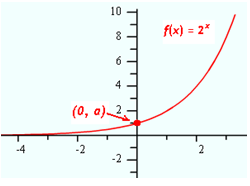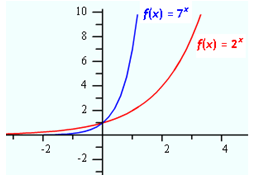Exponential Curves

The graph of f(x) = 2x is below. The value of a is 1 and b is 2. Notice that the curve intersects the y-axis at 1, or the value of a, and that the curve is increasing from left to right. The curve approaches but never intersects the x-axis, so the x-axis is an asymptote of the curve.The curve above is an example when the value of b is greater than 1. What happens to the curve as the value of b increases? The graph below shows how the curve changes as the value of b increases. Notice that the y-intercept remains the same, but the curve gets steeper from left to right.Listen